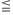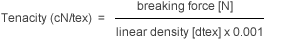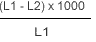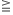Swicofil yarn test methodsManual Overview Twist Directions Yarn Numbering Properties Table Test Methods Monofil diam. conversion Fiber chart Other Conversions Count Converter

1. Test room conditions

The standard according to BISFA are:
- temperature: 20 ± 2°C
- relative humidity: 65 ± 2%

All the tests must be carried out in a conditioned state.

2. Linear density

The linear density decitex [dtex ] corresponds to the yarn weight in grams of 10'000 m length.

In order to determine the linear density, a sample of 25 or 100 meters length is wound on a standard reel under a pretension of 0.5 cN/tex and weighed on an analytical scale. Hereafter, the grams per 10'000 m yarn are calculated.

3. Breaking force and elongation at break

The breaking force leads to the rupture of the thread or yarn. The corresponding value is Newton [N ] .

The elongation at break is the increase in length produced by stretching a test specimen to the breaking point, expressed as a percentage of the initial length. The test is carried out on a dynamometer with a constant increase in elongation, according to the following conditions:

- length between clamps 500 mm (or 250 mm)
- Test velocity; if elongation at break:8%50 mm/min / (25mm/min)
8-50%   500 mm/min / (250 mm/min)
>50%    1000 mm/min / (500 mm/min)
- pretension: 0.5 cN/tex

4. Tenacity (yarns count tenacity)

This is calculated from the breaking force and linear density and is expressed in centi Newton per tex [cN/tex ].5. Force at specified elongation (FASE)

This is the force resulting from a defined extension (usually at 2% and 5%).

6. Hot air shrinkage

This is the shrinkage in length in percent caused by treatment with hot air. We distinguish between free shrinkage and shrinkage under pretension.

a) Free shrinkage
This value defines the change of length after the influence of heat.

Method: After the measurement of length (L1) under 1 cN/tex pretension the samples will be treated without pretension at the defined temperature (between 100°C - 200°C) during 15 minutes. After a cooling time of 1 hour under standard atmospheric conditions the shrunken length (L2) will be determined again with 1 cN/tex pretension. The residual shrinkage is then calculated according to the following formula:b) Shrinkage under pretension
This value defines the change of length during the influence of heat.

Method: The sample is introduced into the heating channel with a pretension of  0.1 cN/tex at 180°C. The shrinkage will be read in percent directly from a scale.

 - Polyamide 6 15 min at 180°C - Polyamide 6.6 10 min at 180°C < dtex 940 15 min at 180°Cdtex940 - Polyester 15 min at 180°C

c) Shrinkage curve
The shrinkage curve in our brochure "product information" was obtained by continuous heating under pretension of 0.1 cN/tex. Due to this there can be differences to the indicated value in the table.

7. Hot air shrinkage force

This is the shrinkage force in cN resulting by hot air treatment (180°C).
Pretension 0.1 cN/tex.

8. Hot water shrinkage 95°C

This is the shrinkage in length in percent which is caused by hot water. It is calculated as follows:

- On a standard reel, skeins of 1 m circumference and 5'000 dtex approximately are prepared and measured at a pretension of 1 cN/tex lengthwise (L1).

- The skeins are then treated without tension during 15 minutes in hot water (95°C), dried and conditioned at standard conditions.

- The shrunk length (L2) is determined again with 1 cN/tex pretension.

- The hot water shrinkage in percent is calculated according to the following formula: